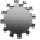Plenary Lecture

Some Implications of a Scale Invariant Model of Statistical Mechanics to Classical and Relativistic ThermodynamicsProfessor Siavash H. Sohrab
Robert McCormick School of Engineering and Applied Science
Department of Mechanical Engineering
Northwestern University
USA
E-mail: s-sohrab@northwestern.edu

Abstract: Some implications of a scale invariant model of statistical mechanics to the mechanical theory of heat of Helmholtz and Clausius will be described. Invariant definitions of reversible heat and work are introduced leading to invariant forms of the first and second laws of thermodynamics. Modified invariant definitions of heat and entropy are presented along with invariant Planck law of equilibrium energy spectrum thus closing the gap between radiation and gas theory. The volume transformation V = Vo(1-β2)1/2 and the modified transformation of relativistic pressure p = po/(1-β2) lead to an ideal gas law that is no longer relativistically invariant pV = poVo/(1-β2)1/2 with β = v/c. The total thermal energy at equilibrium Q is identified as enthalpy H with the relativistic transformation Q = H = U + PV = Qo / (1-β2)1/2 = Ho /(1-β2)1/2. Also, Mie’s theory of matter is discussed and it is shown that the total energy μoc2 = ¾μoc2 + ¼μoc2 of spatially finite universe is three-quarters electromagnetic (dark energy) and one-quarter gravitational (dark matter) in origin in accordance with the ideas of Pauli (Pauli, W., Theory of Relativity, Dover, 1958).

Brief Biography of the Speaker: Siavash H. Sohrab received his PhD in Engineering Physics in 1981 from University of California, San Diego, his MS degree in Mechanical Engineering from San Jose State University in 1975, and his BS degree in Mechanical Engineering from the University of California, Davis in 1973. He joined Northwestern University in 1982 and since 1990 he is Associate Professor of Mechanical Engineering at Northwestern University.